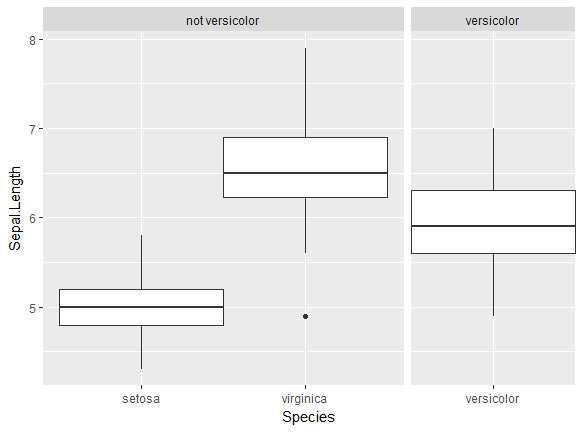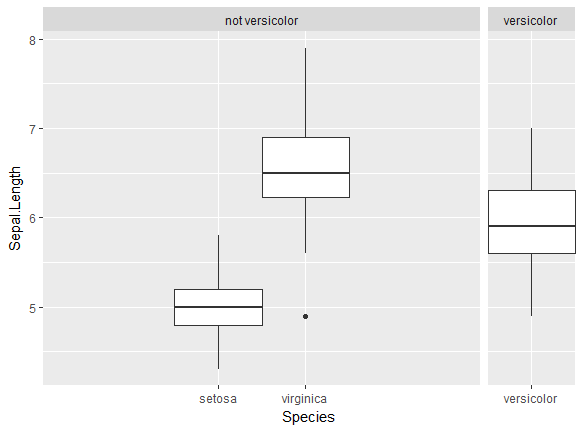/ ggplot2 Public

# Additive constant in `expand=` behaves unexpectedly on discrete axes in facets with one group#2281

Closed
opened this issue Oct 4, 2017 · 4 comments · Fixed by r-lib/scales#161
Closed

# Additive constant in `expand=` behaves unexpectedly on discrete axes in facets with one group #2281

opened this issue Oct 4, 2017 · 4 comments · Fixed by r-lib/scales#161
Labels
bug an unexpected problem or unintended behavior facets 💎

### brianwdavis commented Oct 4, 2017

 This issue occurs when using a discrete axis on in a faceted plot with free scales and free space, and one of the panels only has a single break on the axis. I would expect that the physical space taken up by each tick is the same (it is), but also that the `expand = ` argument would behave independently of the number of ticks on the axis (it does not). This means that the physical space outside the ticks increases for panels with multiple ticks, but it is not changing for panels with only one tick. ```library(ggplot2) library(dplyr) library(forcats) iris %>% mutate(S2 = fct_collapse(Species, "not versicolor" = c("setosa", "virginica"))) %>% ggplot(aes(Species, Sepal.Length)) + geom_boxplot(width = 1) + facet_grid(~S2, scales = "free_x", space = "free_x") + scale_x_discrete(expand = c(0, 0.6)) # Note the default `expand = c(0, 0.6)` ```Here the boxes touch the edges of the panel in the versicolor facet, but there is an expansion gap in the not versicolor facet. This seems to not be expected behavior, since the space for 0.6 of an extra tick should be there in both panels. If you try to increase the additive constant to add an expansion gap in the versicolor facet, no gap is created: ``````iris %>% mutate(S2 = fct_collapse(Species, "not versicolor" = c("setosa", "virginica"))) %>% ggplot(aes(Species, Sepal.Length)) + geom_boxplot(width = 1) + facet_grid(~S2, scales = "free_x", space = "free_x") + scale_x_discrete(expand = c(0, 2)) # Note additive constant increased from 0.6 to 2 ``````Space for a full discrete break was added on each side of the not versicolor facet, but no space was added to the sides of the versicolor facet, so the edges of the box still touch the edge of the panel. The text was updated successfully, but these errors were encountered:

### hadley commented Nov 14, 2017

 The root cause of the problem is `scales::zero_range()`: if the scale has a range of zero it always uses `c(range - zero_width / 2, range + zero_width / 2)`, which ignores the `mul` and `add` parameters.

### hadley commented Jul 24, 2018

 /move to r-lib/scales

### dpseidel commented Jul 26, 2018

So this turns out to be kind of a two part problem. The first part, that `mul` and `add` parameters were not respected for scales of range = 0 is fixed by r-lib/scales#161 which will be merged shortly. I suggest that PR close this issue.

# The fix:

With the new PR, the motivating problem is fixed because the range is now calculated to allow for the default `add = .6` and is thus slightly wider

```library(ggplot2) ### loading scales PR161
library(dplyr)
library(forcats)
iris %>%
mutate(S2 = fct_collapse(Species, "not versicolor" = c("setosa", "virginica"))) %>%
ggplot(aes(Species, Sepal.Length)) +
geom_boxplot(width = 1) +
facet_grid(~S2, scales = "free_x", space = "free_x")``````# scales PR161
iris %>%
mutate(S2 = fct_collapse(Species, "not versicolor" = c("setosa", "virginica"))) %>%
ggplot(aes(Species, Sepal.Length)) +
geom_boxplot(width = 1) +
facet_grid(~S2, scales = "free_x", space = "free_x") +
scale_x_discrete(expand = c(0, 2))```# The still broken? A new issue...

However, I can still create this issue and more! The second part of our two part bug has to do with how the `width` parameter in `geom_boxplot()` (and associated geoms), plots. This will need to be addressed in a separate issue but I'll give a brief overview here:

In the original post, `width` is set equal to 1 in `geom_boxplot()` and the lone boxplot fills the entire facet range set by the `c(range - zero_width / 2, range + zero_width / 2)` because zero_width also equals 1. This is fixed by the new version, but only in the sense that the width is now by default larger (1.2) and adjustable by `mul` and `add`. Even with the new scales fix, if I ask for a `width` ≥ 1.2 without adding to the expand argument, my boxplot will touch the edge of the facet in the single facet plot.

```# scales PR161
iris %>%
mutate(S2 = fct_collapse(Species, "not versicolor" = c("setosa", "virginica"))) %>%
ggplot(aes(Species, Sepal.Length)) +
geom_boxplot(width = 1.5) +
facet_grid(~S2, scales = "free_x", space = "free_x")```But thankfully now the add argument can help mediate this manually.

```# dev scales PR 161 again
iris %>%
mutate(S2 = fct_collapse(Species, "not versicolor" = c("setosa", "virginica"))) %>%
ggplot(aes(Species, Sepal.Length)) +
geom_boxplot(width = 1.5) +
facet_grid(~S2, scales = "free_x", space = "free_x") +
scale_x_discrete(expand = c(0, 2))```However, looking at both the above plots, you'll notice that the width of the boxplots in the first facet is actually smaller (not larger as specified) and they no longer line up with their ticks. This is not a result of the new PR (I've triple checked) but rather a wholly different bug I've found in how `width` is handled for these geoms when a user specifies a large value.

This is especially evident for groups of more boxplots, made with scales 0.5.0 and ggplot2 3.0.0:

``````diamonds %>%
mutate(S2 = fct_collapse(clarity, "not vs2" = c("I1", "SI2", "SI1", "IF", "VS1", "VVS1", "VVS2"))) %>%
ggplot(aes(clarity, price)) +
geom_boxplot(width = 2) +
facet_grid(~S2, scales = "free_x", space = "free_x")
``````Interestingly the behaviour is very erratic depending on `width` value. I'm not yet sure what is causing this separate bug (TBD) but I suggest that this original issue should be closed when r-lib/scales#161 is merged and a new issue opened to investigate the behavior of the `width` parameter which incidentally is not typically user specified, instead calculated internally.

pushed a commit to r-lib/scales that referenced this issue Jul 26, 2018
``` Adjust expand_range() so zero range scales respect mul and add (#161) ```
``` ddfb517 ```
`fixes tidyverse/ggplot2#2281`

### lock bot commented Jan 22, 2019

 This old issue has been automatically locked. If you believe you have found a related problem, please file a new issue (with reprex) and link to this issue. https://reprex.tidyverse.org/